Next: 2.3 Mixture of local Up: 2.2 Vector quantization Previous: 2.2.3 Deterministic annealing

## 2.2.4 Neural Gas

Martinetz et al. (1993) presented an algorithm called `Neural Gas' that outperforms deterministic annealing (Rose et al., 1990). Neural Gas is also a variant of soft-clustering, and it also uses annealing. In contrast, Neural Gas has a different (heuristic) assignment, and it can be only carried out as an on-line version.

The algorithm starts by randomly choosing m data points as starting points for the m code-book vectors. The annealing consists of a predefined number of tmax steps. During each annealing step t, a pattern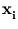is randomly drawn from the training set. Then, the code-book vectors are sorted in the order of their Euclidean distance to. Let k(,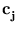(t)) be the resulting rank of each code-book vector, with k = 0 for the closest vector, and k = m - 1 for the most distant vector. The soft-assignment is then given by the function h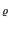ij = exp(- k(,(t))/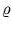). The parameteris a measure of the neighborhood range. Given this assignment, all code-book vectorsare updated according to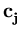(t + 1) =(t) +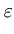(t)h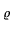(t)ij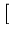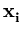-(t)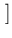. (2.15)

During the annealing process both parameters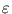anddecrease exponentially,(t) =(0)[(tmax)/(0)](t/tmax), and(t) =(0)[(tmax)/(0)](t/tmax). At the end of the annealing, the algorithm changes to on-line k-means, and thus, also (locally) minimizes (2.12). The exponential decay ofenforces convergence. Neural Gas is stable and does not depend on the initial configuration of {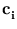} (Martinetz et al., 1993).Next: 2.3 Mixture of local Up: 2.2 Vector quantization Previous: 2.2.3 Deterministic annealing
Heiko Hoffmann
2005-03-22